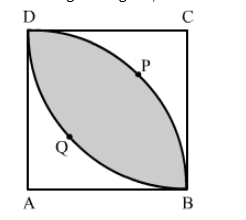# In the given figure, ABCD is a square of side 7 cm, DPBA and DQBC are quadrants of circles each of the radius 7 cm.`
Question:

In the given figure, ABCD is a square of side 7 cm, DPBA and DQBC are quadrants of circles each of the radius 7 cm. Find the area of shaded region.Solution:

Area of the shaded portion = (Area of quadrant DPBA + Area of quadrant DQBC) − Area of Square ABCD

$=\left[\frac{1}{4} \pi(7)^{2}+\frac{1}{4} \pi(7)^{2}\right]-(7)^{2}$

$=\frac{1}{2} \times \frac{22}{7}(7)^{2}-49$

$=28 \mathrm{~cm}^{2}$

Hence, the area of the shaded portion is 28 cm2.